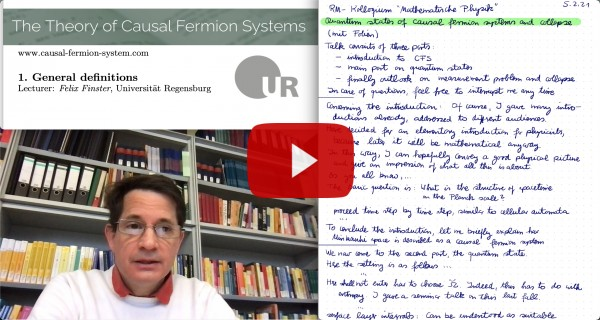# The Theory of Causal Fermion Systems

## Basic DefinitionsDefinition. Given a separable complex Hilbert space $\mathscr{H}$ with scalar product $\la .|. \ra_\H$ and a parameter $n \in \N$ (the spin dimension), we let $\F \subset \Lin(\H)$ be the set of all self-adjoint operators on $\H$ of finite rank, which (counting multiplicities) have at most $n$ positive and at most $n$ negative eigenvalues.
On $\F$ we are given a positive measure $\rho$ (defined on a $\sigma$-algebra of subsets of $\F$), the so-called
universal measure. We refer to $(\H, \F, \rho)$ as a causal fermion system.

In order to single out the physically admissible causal fermion systems, one must formulate physical equations. To this end, we impose that the universal measure should be a minimizer of the causal action principle, which we now introduce. For any $x, y \in \F$, the product $x y$ is an operator of rank at most $2n$. We denote its non-trivial eigenvalues counting algebraic multiplicities by $\lambda^{xy}_1, \ldots, \lambda^{xy}_{2n} \in \C$ (more specifically, denoting the rank of $xy$ by $k \leq 2n$, we choose $\lambda^{xy}_1, \ldots, \lambda^{xy}_{k}$ as all the non-zero eigenvalues and set $\lambda^{xy}_{k+1}, \ldots, \lambda^{xy}_{2n}=0$). We introduce the spectral weight $| \,.\, |$ of an operator as the sum of the absolute values of its eigenvalues. In particular, the spectral weights of the operator products $xy$ and $(xy)^2$ are defined by

$|xy| = \sum_{i=1}^{2n} \big| \lambda^{xy}_i \big| \qquad \text{and} \qquad \big| (xy)^2 \big| = \sum_{i=1}^{2n} \big| \lambda^{xy}_i \big|^2 \:.$

We introduce the Lagrangian $\L$ and the causal action $\Sact$ by

\begin{align}
\L(x,y) &= \big| (xy)^2 \big| – \frac{1}{2n}\: |xy|^2 \\
\Sact(\rho) &= \iint_{\F \times \F} \L(x,y)\: d\rho(x)\, d\rho(y) \:.
\end{align}

The causal action principle is to minimize $\Sact$ by varying the universal measure under the following constraints,

volume constraint:                                          $\rho(\F) = \text{const}$
trace constraint:                             $\displaystyle \int_\F \tr(x)\: d\rho(x) = \text{const}$
boundedness constraint:   $\displaystyle \T(\rho) := \iint_{\F \times \F} |xy|^2\: d\rho(x)\, d\rho(y) \leq C$,

where $C$ is a given parameter (and $\tr$ denotes the trace of a linear operator on $\H$).

→ generalizations and special cases
→ existence theory
→ Euler-Lagrange equations
→ Example: Describing Minkowski space as a causal fermion system

## Causal Structure

We define spacetime as the support of the universal measure,

spacetime $M:= \text{supp} \,\rho$

The fact that the eigenvalues of the above operator products are in general complex gives rise to the following “spectral” definition of the causal structure.

Definition (causal structure). Two points $x, y \in M$ are called spacelike separated if all the $\lambda^{xy}_j$ have the same absolute value. They are said to be timelike separated if the $\lambda^{xy}_j$ are all real and do not all have the same absolute value. In all other cases (i.e if the $\lambda^{xy}_j$ are not all real and do not all have the same absolute value), the points $x$ and $y$ are said to be lightlike separated.

According to the above definitions, points with spacelike separation drop out of the causal Lagrangian. This can be seen in analogy to the usual notion of causality where points with spacelike separation cannot influence each other. This analogy is the reason for the notion causal in “causal fermion system” and “causal action principle.”

## Other Inherent Structures

Apart from the causal structure, a causal fermion system gives rise to many additional structures. These structures are all inherent in the sense that they use information already encoded in the causal fermion system.